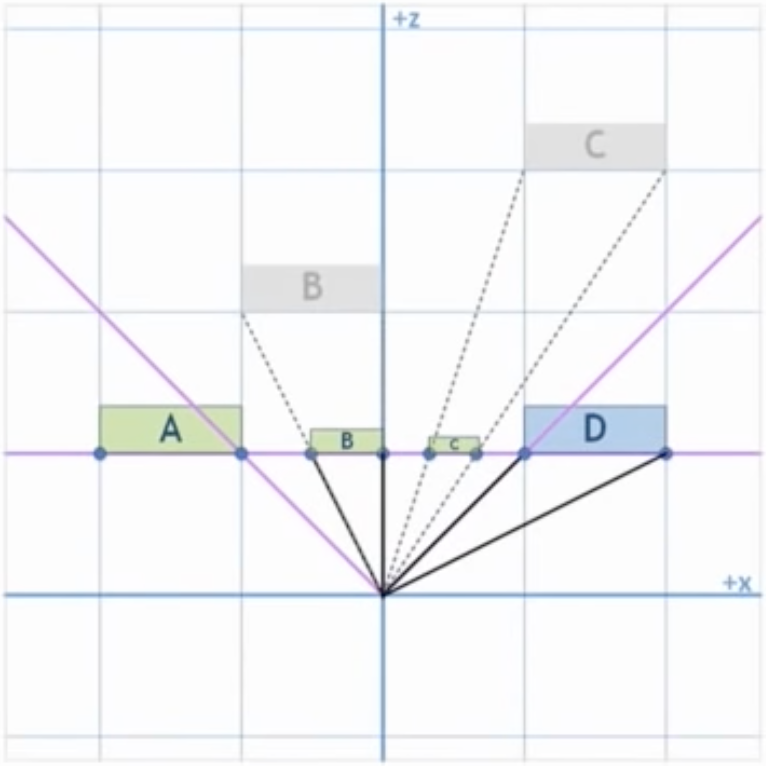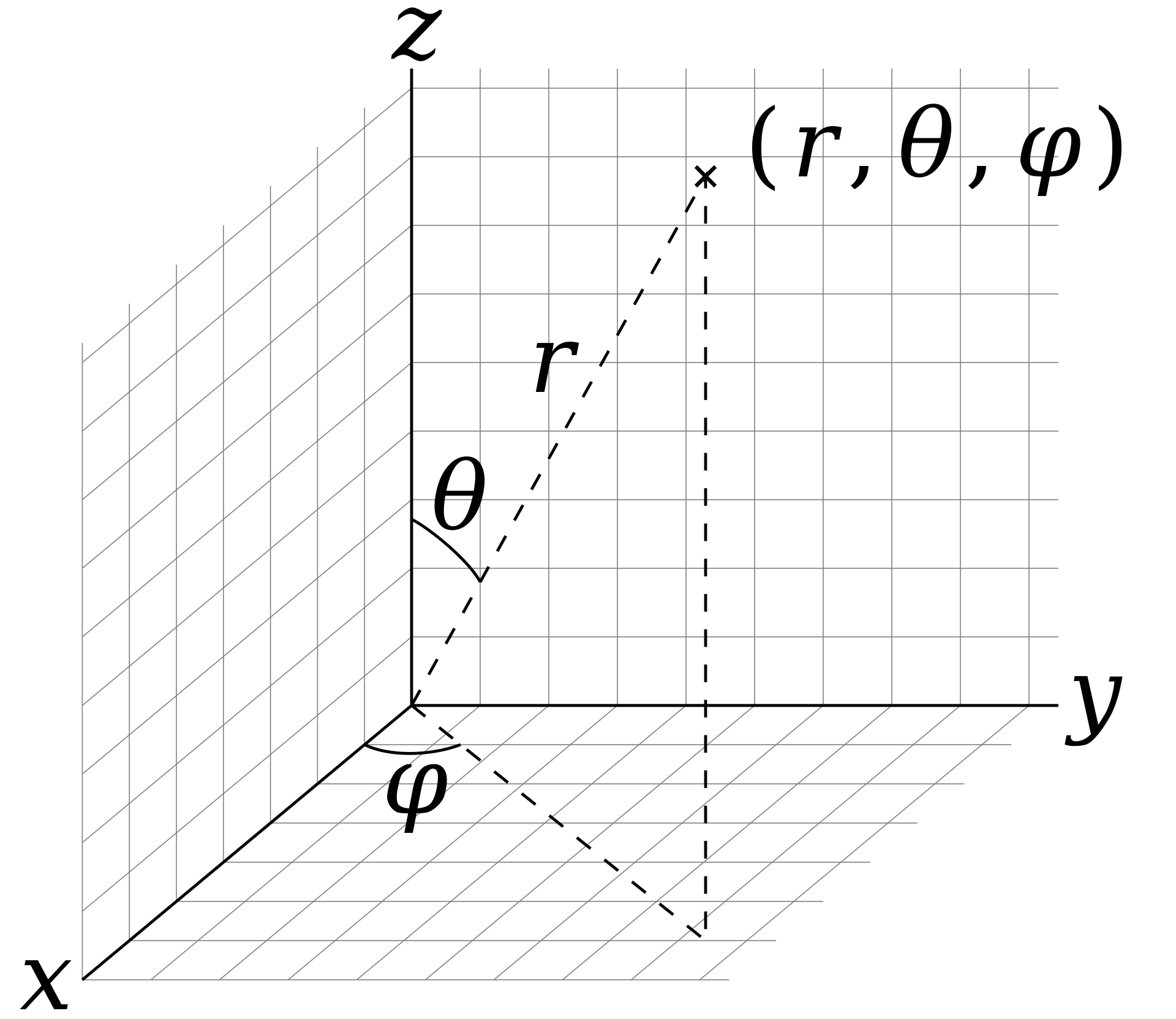## Homogenous §

We add a new coordinate (sometimes also ). To convert homogenous coordinates back into Cartesian coordinates, we divide through by :

### As groundedness §

If we interpret as groundedness, it measures how ‘translateable’ it is:

• for directions/vectors
• for positions
• need to be divided by to come back into real space

### As depth §

We can also interpret as camera depth.

What makes something look 3D?

• Relative size (far objects are smaller)
• Perspective (far objects converge on the origin)
• Motion parallax (far objects move slowly)

Dividing through gives us these three effects.

In order to make distant objects appear smaller and closer to the center of the screen, we use a coordinate system with at the center of the screen (this is done in the CCS).

This effectively projects onto the ‘near’ plane## Barycentric Coordinates §

Mostly used for interpolation. Given values known at the vertices (e.g. depth, colour, texture, surface normals), we wish to linearly interpolate their values for the interior pixels

Barycentric coordinates can be seen as a weighted combination of vertices.

To interpolate,

We use barycentric coordinates instead of screen space coordinates because the mid-point in screen-space may not be the midpoints along the real line/object.

Calculating the weighted value for the vertex :

1. Write an explicit line equation for the edge opposite the vertex
2. Convert it into an implicit line equation ( in this example, line connecting and )
3. Evaluate the implicit line equation at the vertex, let this be

## Spherical §

represents the polar angle (angle from the vertical axis) and represents the azimuthal angle (angle from the axis)